Home > MC1 > Chapter 8 > Lesson 8.2.3 > Problem8-53

8-53.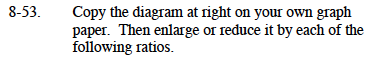If we use each ratio as new/original, then the example below shows the lengths of a new diagram that would have sides that are five times as long as the original.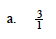If you choose to use a ratio of new/original, you will need to enlarge the figure. Use the example.
Can you draw your own diagram?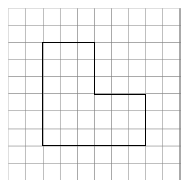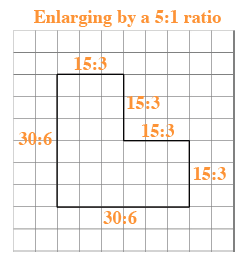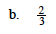$\text{For part (b), the new diagram will be }\frac{2}{3}\text{ the size of the original.}$

$\text{Since all sides of the figure are 3 units or 6 units, it may help to know that }\frac{2}{3} \text{ of 3 is 2 and }\frac{2}{3}\text{ of 6 is 4.}$

Can you draw the new figure?